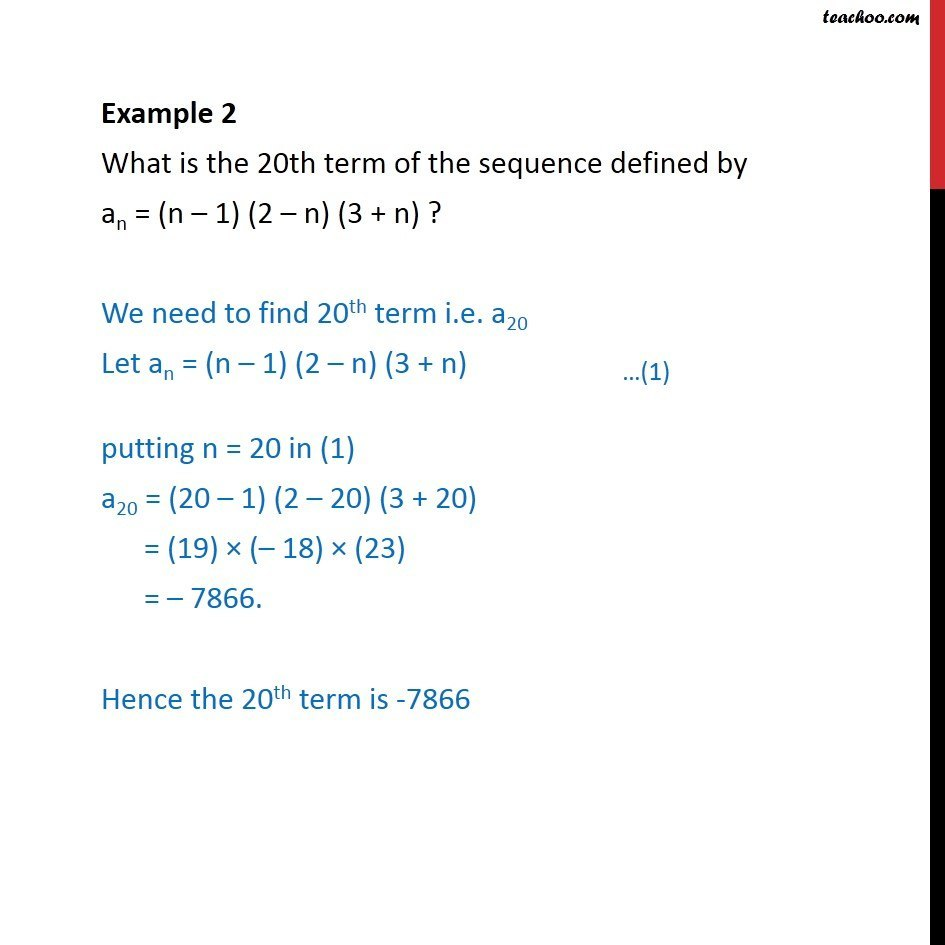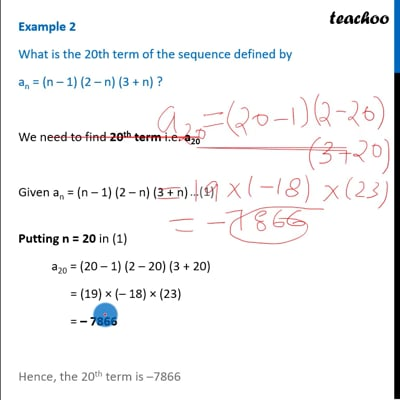Examples

Chapter 9 Class 11 Sequences and Series
Serial order wiseThis video is only available for Teachoo black users

Solve all your doubts with Teachoo Black (new monthly pack available now!)

### Transcript

Example 2 What is the 20th term of the sequence defined by an = (n 1) (2 n) (3 + n) ? We need to find 20th term i.e. a20 Let an = (n 1) (2 n) (3 + n) putting n = 20 in (1) a20 = (20 1) (2 20) (3 + 20) = (19) ( 18) (23) = 7866. Hence the 20th term is -7866